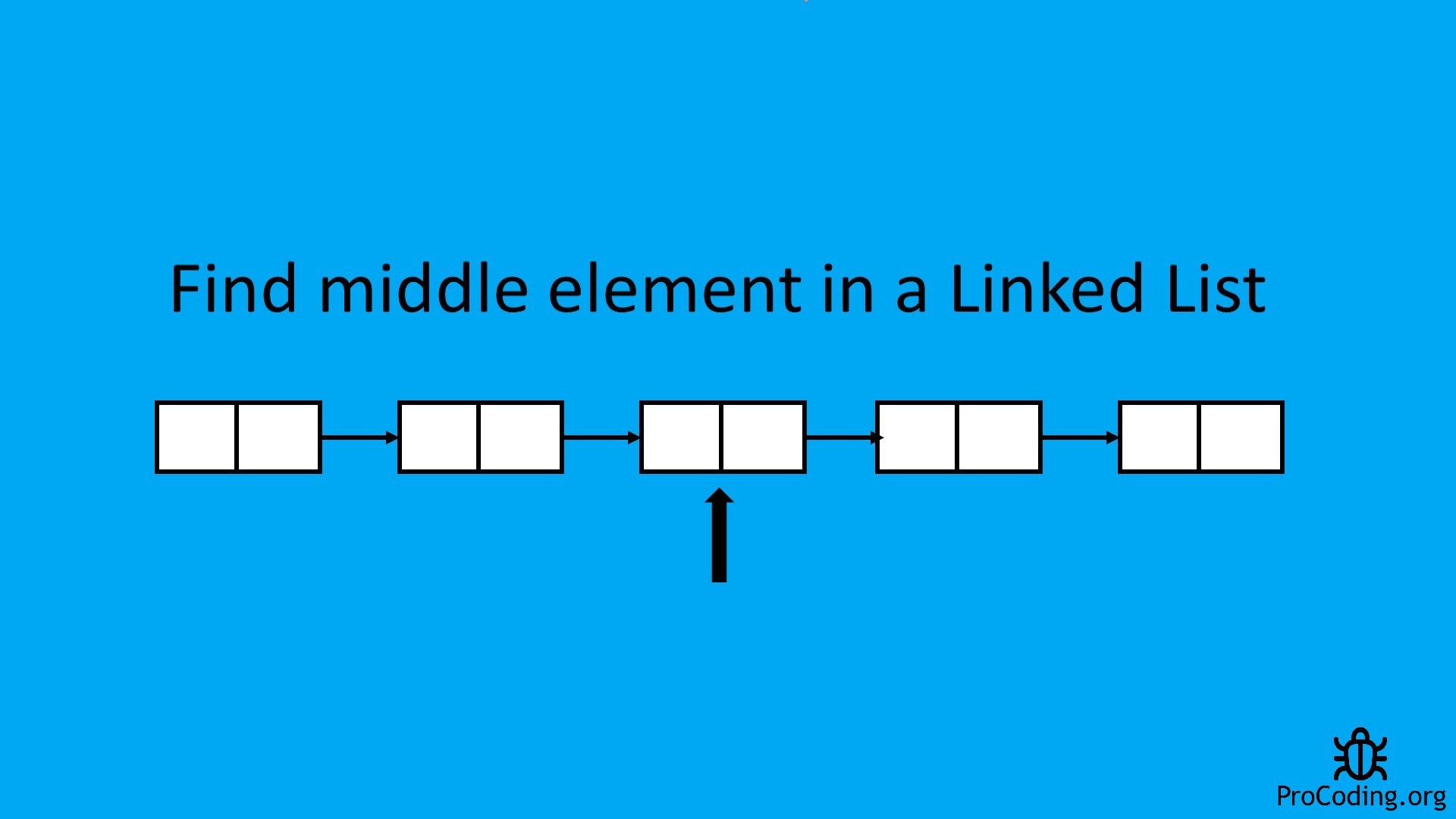# Program to find middle element of a Linked List

Abhishek Kumar Category: Data StructuresTags: datastructures linkedlist

Given a linked list, write a program to find middle element of a linked list.
e.g.- for linked list 1 -> 2 -> 3 -> 4 -> 5, output should be 3.#### Problem-

Find the middle or centre element in a singly list.

#### Solutions-

Solution 1: We can use the naive approach by simply traversing the linked list and count the number of elements then divide the number of elements by 2.

Then go for a second loop and traverse for the half of the number of elements in the linked list. But in this approach, we have to use two loops for iterating over the linked list twice.

Solution 2: We may go for another solution which is to use two pointers on the linked list. The first pointer will be a slow pointer i.e., moves one step for every iteration and the second pointer will be the fast pointer which moves two steps for every iteration.

In the end, the linked list will be divided into parts and the fast pointer will be at the end of the linked list and the slow pointer will be at the middle of the linked list. We can achieve this by using one loop only. In this post, we will this approach to solve the problem.

#### Program

``````class Node:
def __init__(self, data):
self.data = data
self.next = None

def __init__(self):

def insertAtEnd(self, data):
node = Node(data)
else:
while temp.next:
temp = temp.next
temp.next = node

def traverse(self):
while temp:
print(temp.data, end=' ')
temp = temp.next
print()

def findCenterNode(self):
while fastPtr and fastPtr.next:
fastPtr = fastPtr.next.next
# fastPtr = fastPtr.next
ptr = ptr.next
print(ptr.data)

print('Middle element is: ')
``````class Node {
int data;
Node next;
Node(int data) {
this.data = data;
this.next = null;
}
}

}

public void insertAtEnd(int data) {
}
else {
while (temp.next != null) {
temp = temp.next;
}
temp.next = new Node(data);
}
}

public void traverse() {
while (temp != null) {
System.out.print(temp.data + " ");
temp = temp.next;
}
System.out.println();
}

public void findCenterNode() {
while (fastPtr != null && fastPtr.next != null) {
fastPtr = fastPtr.next.next;
ptr = ptr.next;
}
System.out.println(ptr.data);
}
}

class Main {
public static void main(String[] args) {
ll.insertAtEnd(5);
ll.insertAtEnd(1);
ll.insertAtEnd(6);
ll.insertAtEnd(8);
ll.insertAtEnd(9);
ll.insertAtEnd(4);
ll.insertAtEnd(2);
System.out.println("Middle element is: ");
ll.findCenterNode();
}
}``````

#### Output

Output

```Middle element is:
8```

AUTHOR### Abhishek Kumar

Software engineer | Blogger | Keen learner
Python | Django | JavaScript | React | Next.js | Express.js | C
Passionate about learning and experimenting with new things and open to more opportunities and collaborations.